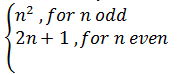# A mapping f : n -> N, where N is the set of natural numbers is defined as f (n) =for n belongs to N. Then f is (1) surjective but not injective (2) injective but not surjective (3) bijective (4) neither injective or not surjective

QuestionSolution: (4)

f (n) =n, n2 and 2n+1 is odd ∀ n ∈ N

f (n) is not even.

⇒ It is not onto or not surjective.

Since, f (3) = f (4), f (n) is neither one-one nor injective.

So, f (n) is neither injective nor not surjective.(0)(0)